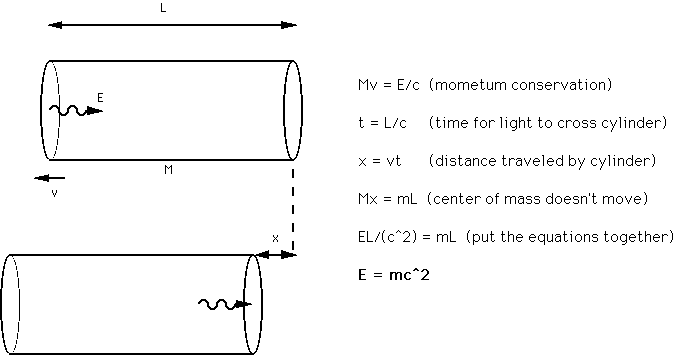"Proof" of E=mc2

Before Einstein, it was known that a beam of light pushes against matter; this is known as radiation pressure. This means the light has momentum. A beam of light of energy E has momentum E/c. Einstein used this fact to show that radiation (light) energy has an equivalent mass.Consider a cylinder of mass M (see accompanying figure-"energy"). A pulse of light with energy E is emitted from the left side. The cylinder recoils to the left with velocity v=E/(Mc). If the mass of the cylinder is large, it doesn't move far before the light reaches the other side. So, the light must travel a distance L, requiring time t=L/c. In this time, the cylinder travels a distance x=vt=[E/(Mc)](L/c).

Pause the movie by clicking on the || button on the left.

Einstein reasoned that the center of mass of an isolated system doesn't just move on its own. So, the motion of the cylinder must be compensated by the motion of some other mass. Let's assume the light has mass m. Then, Mx=mL, since the cylinder moves x to the left and the light moves L to the right. Substituting the expression for x given above, the equation can be simplified to E=mc2.

From the fact that light has momentum, Einstein showed that light energy has the characteristics of mass also. In other words, energy has inertia. It turns out that all energy has this feature. That's because one form of energy can be transformed into another. So, if one kind of energy has this characteristic, all forms of energy do.

Einstein himself explains the meaning of E=mc2 in this sound clip.

The fine print: The word proof is in quotes above because this is not truly a rigorous proof. Simplifications and approximations were made to facilitate understanding. Some of these are easy to eliminate at the expense of a little more algebra. Some of them are of a more fundamental nature and require significant modification of the gedankenexperiment. However, the basic concepts are correct and this "proof" conveys the essence of the connection between mass and energy.

This page contains QuickTime movies. QuickTime is available for Windows and MacOS.